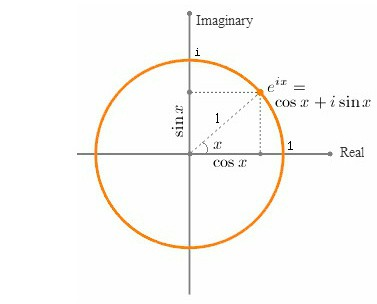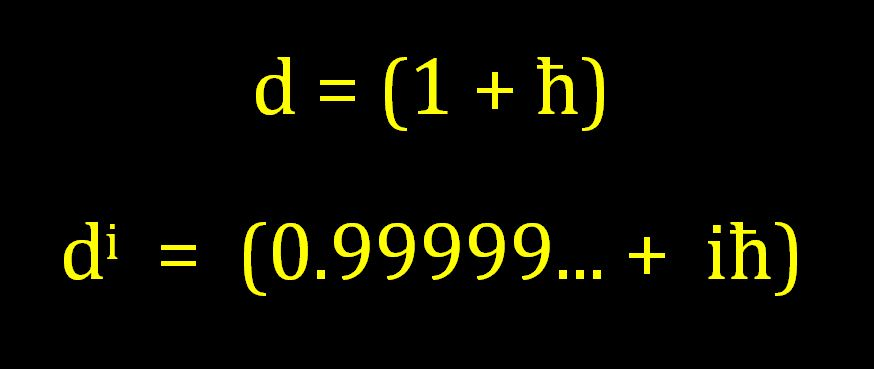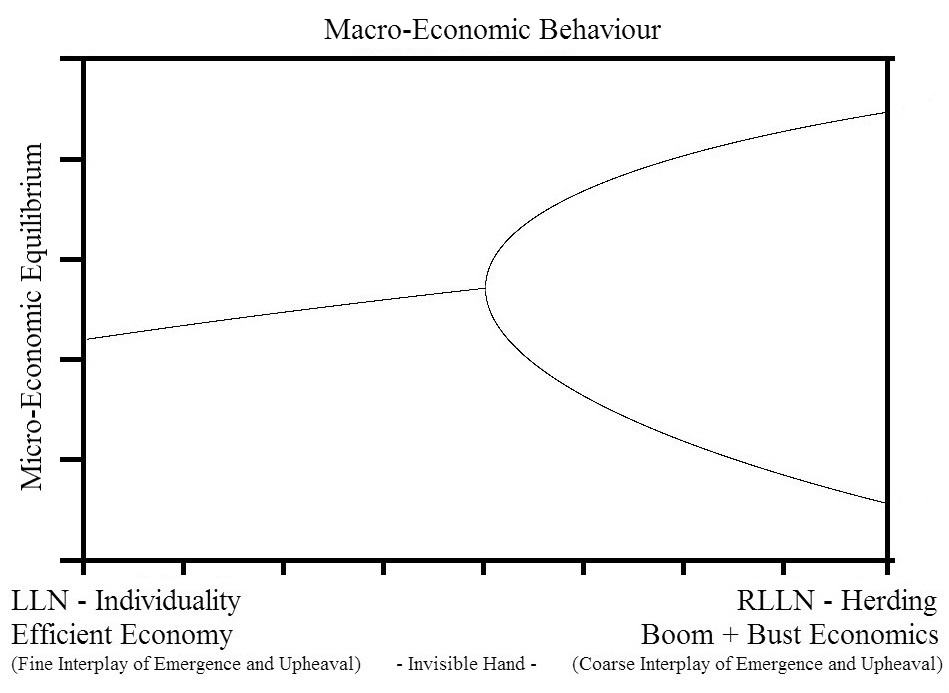# Blog

## What is Euler’s Secret Identity?Is there some connection between the Mathematics of Euler’s Identity and the Physics of a Photon?

Euler’s Formula (eix = cos(x) + i sin(x) ) is usually thought of as a mathematical description of how a “vector rotates” through an angle in the complex plane; and consequently Euler’s Identity is usually considered simply a rotation through an angle of π radians.  But as long ago as 1581 Galileo had already realized something amazing; “rotation” has a secret identity — he is also an “oscillation”…

Galileo’s startling realization that uniform circular motion and periodic motion were different sides of the same coin, meant that he saw that angles could also be used to describe an oscillation.  And this, it turns out, was a truly brilliant insight!…

So although most people would probably still associate angles with geometry and trigonometry, angles can also be associated with the amount of “energy in an oscillation” –-  which means that a function that defines an amount of  rotation in the complex-plane ( y=(-1+i0))  can also be thought of as a “definable angular frequency” expressed as x% of (-1+i0), or in other words: “ x% of a half-cycle”.

Now, what is really interesting about this idea is that:  the amplitude of all “normalized” oscillations have a magnitude of 0.25 units of measurement, or ¼ of the normalized cycle.  So

• In our formula ( y=(-1+i0)), when we set  x=0.5, we get “a quarter-rotation in the complex plane”

(-1+i0)0.5   =   (0+i1)

• But in setting x=0.5 we are also, in effect, setting an angular frequency of π/2 radians for the equivalent oscillation on the real-line; and so we could also say that (0+i1) is “a quarter-oscillation on the real-line”, which means that

(0+i) is the amplitude of all normalized oscillations

• And this means that

(-1) is the “amplitude of an normalized oscillation squared (i2)”

• And this means that

all frequencies can be defined as some % of (i2)

=======

So, any angular frequency of oscillation, defined in terms of a single half-cycle ( y = (-1)x ), can now also be defined in terms of the normalized amplitude squared  ( y = (i2)x ).  And this, to anyone who has studied even a small amount of physics is strangely reminiscent of the “energy in simple harmonic motion”.

This would seem to suggest that an angular frequency (i2)x  might be equivalent is some way to the energy in an oscillation (½kA2), and that (x) might represent some form of “restoring variable”.

Furthermore since we know that the equation for the energy in a photon of light is

E  =  hf  =  ħω

This further suggests that there might be some form of a connection between (eiπx) and (ħω).  So let’s take a closer look at ( ei )…

We can use Euler’s Formula to define a smallest “unit of angular change”.  Let’s define this unit to have the same magnitude as the value of Planck’s Reduced Constant.eix   =   =   cos(x) +  i sin(x)

ei   =   cos(1) +  i sin(1)

eiħ   =   cos(ħ) +  i sin(ħ)

(-1)ħ   =   (0.99999999 +  iħ)

Given this defined “unit of angular change”, we can now think of every other size of angular change as an integer number(n) of units of (1 +iħ);

(eiħ)n   =   (ei)ħn   =   einħ   =   (1 + iħ)n   =   n ħ-radians   (where n = 1,2,3….)

And when n = Ħ =  9.48252238653324  x  10+33 (i.e. capital-h-bar : the inverse of  ħ) we get an angle of

(eiħ)Ħ   =   (ei)ħĦ   =   ei   =   (1 + iħ)Ħ   =   Ħ ħ-radians =   1 radian

And when n = Ħπ we get an angle of

(eiħ)Ħπ   =   (ei)ħĦπ   =   eiπ   =   (1 + iħ)Ħπ   =   π radians  = (-1+i0)So if (-1)x  is indeed equivalent to  (½kA2) we could now say that since

(-1)x   =   (eiπ)x   =   (1+ iħ) Ħπx

And since

½kA2   =   hf

ThereforeSo maybe different photon frequencies simply represent different amounts of restoring force in the elastic fabric of space-time.  If so then different frequencies would simply represent different percentages of the ultimate elasticity of space-time.

So what is Euler’s Secret Identity?

Maybe he is the speed of light’s cousin;

“The Angular Speed of Mass” – the fastest frequency of all…

Posted on Categories Uncategorized

## How Do You Like Them Apples?Named after the Swiss mathematician Leonhard Euler – Euler’s Identity is often described as an example of deep mathematical beauty.  Some see it almost as proof of the existence of some hidden order of the universe that we can only catch a glimpse of…

This equation is considered amazing because it seems to sets up a relationship between three apparently completely unrelated subjects.

“e” originated from the study of compound interest (and was subsequently found to be the base of the natural logarithms); “π” originated from Geometry and Trigonometry; and i” was “invented” to help with solving equations involving negative numbers.

Compound Interest, Trigonometry, and Imaginary Numbers?…  The question that has intrigued mathematicians and physicists since Euler first published his famous formula in 1748, is “Why in God’s name do these (apparently completely unrelated) areas of mathematics fit together so neatly in this beautiful equation?”

###### Well here’s the answer – Watch!

[Note: What follows is just a quick jump-thru, if you want the in-depth understanding click here]

e  =  Limit as n →  (1 + 1/n)n  =  2.718281828459045……

For practical purposes, “e” is difficult to pin down is simply a consequence of the fact that “e” is a manufactured quantity, defined using “limits to infinity”, and infinity is an idea not a number.

In reality there is no definitive value of “Transcendental” “e” because we can theoretically infinitely add more and more decimal places to the value of “e” by constantly increasing n all the way to infinite (which doesn’t actually exist).

However although the concept of infinity might be vague, “the concept of “e””, on the other hand, is actually all about very finely-grained precision…

The concept and formula for “e” is neat and very precise because the number of steps (n) must be the exact inverse of the tiny step-size (1/n).

In order to discuss the preciseness of “e”, it is better, for the purposes of this discussion, to limit the influence of “infinity” and define “e” with very precise numbers.

To do so, we will use both a very large number and a very small number which are exact inverses of each other.  We will use the symbol Ħ for the very large number, and we will use the symbol ħ for the very small number.

Ħ  =  10,000,000,000,000,000,000,000,000,000,000,000  =  10+34

ħ  =  0.000,000,000,000,000,000,000,000,000,000,000,1  =  10-34

Since ħ = 1/Ħ we can now write a precision equation for “e” as

e = ( 1  +  ħ )Ħ  =  2.718281828459045……

Okay, so now away we go…

e = ( 1  +  ħ )Ħ

e1/Ħ = ( 1  +  ħ )

eħ = ( 1  +  ħ )

======

Set       d  =  eħ  =  ( 1  +  ħ )

======

dxĦ  =  (1 + ħ)xĦ  =  (eħ)xĦ  =  (eħĦ)x  ex

so

d  =  (1 + ħ)1Ħ  (eħ)1Ħ  =  (eħĦ)1  =  e1  =  2.718281828459…

[Ħ micro-steps of (1+ħ) is equivalent to 1 macro-step of 2.71828]

Okay that was for real numbers, now let’s do complex numbers…

(d)i  =  (ex)i  =  cos(x) +  i sin(x)

(d)i  =  ei  =  cos(1) +  i sin(1)

di  =  eħi  =  cos(ħ) +  i sin(ħ)

di  =  (0.99999999… +  iħ)di(xĦ)   =   ( 1 + iħ )xĦ   =   (eiħ)xĦ   =   (eħĦ)ix   =   eix

so

di(1Ħ)   =   ( 1 + iħ )1Ħ   =   (eiħ)1Ħ   =   (eħĦ)i1   =   ei1

[Ħ micro-steps of (1+iħ) is equivalent to 1 macro-step of 1 Radian]

and

di(3.14159…Ħ)  =   ( 1 + iħ )πĦ   =   (eiħ)πĦ   =   (eħĦ)iπ   =   eiπ

[3.14159…Ħ micro-steps of (1+iħ) is equivalent to 1 macro-step of π Radians]

.

di(πĦ)    =    -1.00 + i0.00

⇒     di(πĦ)  =    -1

.

.

How do you like them apples?

So why is any of this important?

It is important because despite what is generally believed, Euler Identity is not telling us something special about “rotations”; it is telling us something special about “oscillations”.

[Remember: this was just a quick jump-thru; for a more detailed explanation click here.]

Euler’s identity is a beautiful example of the “quantized mathematics of change”.This quantization of mathematics allows us to define very precise “coarse” macro step-sizes (both linear and angular) using integer amounts of the quantized micro units d and di.

• d(xĦ) : defines the coarseness of linear growth and decay
• di(xĦ) : defines the coarseness of oscillations[This post is adapted from What is Euler’s Identity?.]

## Long Term Economic Growth

###### “Austerity” or “Quantitative Easing” ?

In nature after an evolutionary collapse, mother-nature will begin to rebuild, and in a normal business cycle so too would an economy.  However in a boom and bust cycle things are not quite so easy!

Disposable Income

Economics is often thought of as a study of the allocation of “scarce” resources.  But that’s just another way of saying that economics is the study of “people making rational decisions”; and unfortunately we now all know that this is not true.

The starting point for all of economics is actually the ability to “produce excess”.  In a time before money, being able to produce more than one’s own immediate needs lead to the emergence of barter.  But with the introduction of money – which acts both, as a means of exchange, and a store of wealth – “excess productivity and production” could lead to the accumulation of “disposable income”; and it is this disposable income that drives an economy in 2 separate ways

1. Consumption: disposable income drives trade, and thus economic activity.
2. Investment: disposable income drives innovation, and thus self-organized, economic-complexity.

[Note: Complexity can be defined as “integrated diversity” and thus a Complex Adaptive Economy is a highly diverse & integrated  system.]

Stagnation

In a boom, investments are funded not only from accumulated savings, but usually also with overly-available credit (i.e non-accumulated future income).  In a bust, investments funded out of future income (i.e. debt) continue to incur ongoing interest costs despite the fall in value (or in many cases wipe-out) of the investment.  Not only that, but unfortunately for the borrower (and the economy at large), the principle also still needs to be repaid.

Thus an economic boom funded by excessive credit, eats into disposable income long after the boom has completely disappeared.  And since disposable income is what drives both investment and consumption, it depletion affects not only the growth of complexity in the economy but also the level of economic activity/trade and thereby the size of the economy.

In an economy the only time we really have stability is when nothing much is happening – and nothing much will happen when the driving force of the “Invisible Hand” is severely weakened.

In a weak economy, with very little funding only the very best ideas have a chance of being funded; but with no funding at all, and no consumer spending to boot, not only is new business growth stunted, but many existing businesses will likely decay! [In an economic bust, even healthy business go under; because a large amount of pre-existing disposable income is been redirected towards paying off debt.]

In a dysfunctional economy, the weight of unproductive debt substantially weakens the driving force of growth and innovation in a normal functional economy.  An economy mired in debt will stagnate!  An economy devoid of disposable income is akin to a planet devoid of sunlight; nothing will grow!

Credit and Excess

Capitalism is perceived as the optimal growth engine of economic development and growth!  Financial Markets are supposed to be the invisible hand that optimally allocates the supply of, and demand for, investment funds in a Complex Adaptive Economy (CAE).  Chaos Theory alerts us however, to a possible vulnerability in the system!

The mathematics of chaos shows us that there is in fact an “Optimal Rate of Investment” in this Driven-Damped System – beyond which the system is vulnerable to “Coarse Synchronicity and Chaos”!

We know that, too much investment can overdrive the system causing the emergence of the so-called “business cycle”; excessive investment however has the effect of converting a functional cycle driven (even if slightly out of sync) by “The Wisdom of Crowds”, finely balancing “Supply and Demand” (of investment funds); into a dysfunctional cycle driven by “The Madness of Mobs” coarsely balancing “Fear and Greed” (of a financial market gamble).  What tips the system from one to the other (i.e. from functional to excessive) is when over-investment becomes “supercharged” by credit-fuelled investment!  Fundamentally, there are 5 levels of Driving Force in a Complex Adaptive Economy

1. Minimum: Stops the natural decay of a naturally damping system.
2. Small Excess: Under-Drives the Economy – a lot of good ideas are left unfunded.
3. Critical Excess: Critically-Drives the Economy – optimally funds all the best ideas.
4. Large Excess: Over-Drives the Economy into a “Business Cycle” – many poor ideas also get funded.
5. Excessive Excess: Supercharges the Economy into a “Boom and Bust and Stagnation-Dead-End” – basically any idea will do!

Clearly credit is the lifeblood of business and trade in the economy.  Credit however has no place in the funding of asset price speculation.  Excessive credit fuelled speculation is simply a build-up of instability in markets; an accident waiting to happen!

LLN + RLLN

Implicit to the idea of the “Wisdom of Crowds” is that no one player in the market might know all the available information, but the market as a whole does.  This apparent “rational wisdom” however, relies heavily on the “Law of Large Numbers” (LLN)!

The LLN is a statistical concept that deals with the idea that statistical accuracy is related to the size of the sample.  Most people would recognize the central thesis: if you toss a coin four times, you won’t necessarily get a 50/50 split of heads and tails: indeed, you could get 4 tails, suggesting (wrongly) that the coin will always land on tails. But if you toss a coin a trillion times, you will get something close to a 50/50 split between heads and tails.

The tendency in financial markets towards “herding behavior” weakens the apparent wisdom of the crowd by reducing the large number of independent players in the market into smaller collective groups, and in so doing, effectively engineers a Reverse of the Law of Large Numbers (RLLN)…Debt and Inequality

The RLLN is the reason why financial markets don’t always do what they are supposed to do.  Financial markets are a Complex Adaptive System and thus inherent vulnerability to the emergence of herding and the RLLN.  If we want these markets to operate in the most efficient fashion, we need to guard against any amplification of the RLLN; we need better control over the amount of credit in the system; both before and after a market bubble.

Vulnerability to the RLLN leads to the sub-optimal allocation of resources.  The misallocation of savings and credit has 4 main effects:

1. In the short term, it burns though savings wasting a valuable economic resource.
2. In the long run, it starves good ideas of investment.
3. It creates inequality, because bad investments always suck the poor in last, allowing the rich to get out…
4. It creates suffocating debt, and with that debt, it sucks the driving force out of the economic system…

Complexity Theory shows us that the “optimal” Complex Adaptive Economy (CAE) self-organizes from the bottom up.  Chaos shows us that there is an optimal rate of investment in a CAE – beyond which financial markets are vulnerable to “coarse synchronicity and chaos”.

Moreover the mathematics of chaos tells us that

In the short term artificially pumped up asset markets, can have a trickle-down effect, but ultimately in the long term, the primary effect is to increase inequality!

The Economic Engine

There has been a long running argument in economics as to whether an economy should be left alone or needs supervision.  In a way both sides are right!

The economy is like a car, it has a battery, but we never use the battery when the car is running – the battery’s sole purpose is to kick-start the car into ignition.  Using excessive credit in a booming economy is always going to flood the engine; lack of credit when the economy is stalled however is like trying to start the car without the ignition.

The takeaway message is that in any Driven-Damped Complex Adaptive System, positive feedback is always required in order for the system to be able to work its way away from the gravitational pull of natural decay.  In the push and pull of a Complex Adaptive Economy, a collapse in positive feedback can collapse the complex equilibrium which can mean that the “Business Cycle” itself effectively disappears.

Excessive Consumer Credit can overdrives an economy, but Excessive Consumer Debt can kill the drive altogether!

We should treat the economy as we would a car – we should leave well alone when the engine is running and the battery is charging; but if the engine is not running, then we have no option but to use the ignition…

=======

But if there is but one single take-home message about understanding the behavior of the Driven-Damped Complex Adaptive Economy for the current economic climate, it is that: a CAE naturally self-organizes “from the bottom up”, driven by “disposable income”, and consequently

“Austerity” severely weakens the driving force of economic growth, and, in such a weaken environment, “Quantitative Easing” will simply widen the inequality gap!

So in answer to the implied headline question, “what will really drive long-term economic growth; austerity or quantitative easing?” – Neither of the above!

[This post is adapted from Incompressible Chaos in Financial Markets.]

Posted on Categories Uncategorized